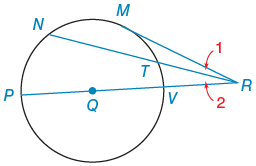Alyssa Z.

# Given:     In ⊙Q, PR contains Q, MR is a tangent, mMP = 116°, mMN = 60° and mMT = 46°.Find the following in degrees.

Consider the following figure.A circle has center point Q. There are six points, three line segments, and two angles.

1. Five points lie on the circle:
2. M is on the top right;
3. N is on the top left;
4. P is on the left, just below center;
5. V is on the right, just above center; and
6. T is on the upper right, between M and V.
7. Point R lies outside the circle, on the right.
8. Segment P R passes through center Q and intersects the circle at V.
9. Segment N R intersects the circle at T.
10. Segment M R is tangent to the circle at M.
11. M R N is labeled 1, and ∠P R N is labeled 2.
 Given:     In ⊙Q, PR contains Q, MR is a tangent, mMP= 116°, mMN= 60° and mMT= 46°. .

Find the following in degrees.

(a) m∠MRP

°

(b) m∠1

°

(c) m∠2

## 2 Answers By Expert Tutors

By:Tutor
4.3 (12)

Math/Science TutorSam Z.

that n is to be an m.
Report

21d

## Still looking for help? Get the right answer, fast.

Get a free answer to a quick problem.
Most questions answered within 4 hours.

#### OR

Choose an expert and meet online. No packages or subscriptions, pay only for the time you need.